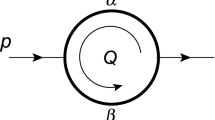# A generalization of simplest number fields and their integral basis

## Abstract

An integral basis of the simplest number fields of degrees 3, 4 and 6 over $$\mathbb{Q}$$ is well-known, and widely investigated. We generalize the simplest number fields to any degree, and show that an integral basis of these fields is repeating periodically.

This is a preview of subscription content, access via your institution.

### Similar content being viewed by others### Mellin–Barnes integrals and the method of brackets

Ivan Gonzalez, Igor Kondrashuk, … Luis M. Recabarren

### A reformulation of the Siegel series and intersection numbers

Sungmun Cho & Takuya Yamauchi

### Multiple expansions of real numbers with digits set $$\left\{ 0,1,q\right\}$$

Karma Dajani, Kan Jiang, … Wenxia Li

## References

1. Cohn, H.: A device for generating fields of even class number. Proc. Amer. Math. Soc. 7, 595–598 (1956)

2. V. Ennola, Cubic number fields with exceptional units, in: Computational Number Theory, Proc. Colloq. Debrecen, 1989 (1991), 103–128

3. Ennola, V.: Fundamental units in a family of cubic fields. J. Théor. Nombres Bordeaux 16, 569–575 (2004)

4. Foster, K.: HT90 and "simplest" number fields. Illinois J. Math. 55, 1621–1655 (2011)

5. I. Gaál, Diophantine Equations and Power Integral Bases. Theory and Algorithms, 2nd ed., Birkhäuser (2019)

6. Gaál, I., Jadrijević, B., Remete, L.: Simplest quartic and simplest sextic Thue equations over imaginary quadratic fields. Int. J. Number Theory 15, 11–27 (2019)

7. Gaál, I., Remete, L.: Integral bases and monogenity of pure fields. J. Number Theory 173, 129–146 (2017)

8. Gaál, I., Remete, L.: Integral bases and monogenity of the simplest sextic fields. Acta Arith. 182, 173–183 (2018)

9. M. N. Gras, Table numérique du nombre de classes et des unités des extensions cycliques réelles de degré 4 de $${\mathbb{Q}}$$, Publ. Math. Fac. Sci. Besançon, Théor. Nombres, 2 (1977-1978), 1–79

10. M. N. Gras, Families of units in real cyclic extensions of $${\mathbb{Q}}$$ of degree 6, Publ. Math. Fac. Sci. Besançon, Théor. Nombres, 1984/85-1985/86 (1986), Exp. No. 2, 27 pp

11. Heuberger, C.: All solutions to Thomas' family of Thue equations over imaginary quadratic number fields. J. Symbolic Comput. 41, 980–998 (2006)

12. Hoshi, A.: On correspondence between solutions of a family of cubic Thue equations andisomorphism classes of the simplest cubic fields. J. Number Theory 131, 2135–2150 (2011)

13. A. Hoshi, On the simplest quartic fields and related Thue equations, in: Computer Mathematics, 9th Asian symposium, ASCM 2009, Fukuoka, Japan, December 14-17, 2009, 10th Asian symposium, ASCM 2012, Beijing, China, October 26-28, 2012, Springer (2014), pp. 67–85

14. Hoshi, A.: On the simplest sextic fields and related Thue equations. Funct. Approx. Comment. Math. 47, 35–49 (2012)

15. Hoshi, A.: Complete solutions to a family of Thue equations of degree 12. J. Théor. Nombres Bordeaux 29, 549–568 (2017)

16. H. K. Kim and J. H. Lee, Evaluation of the Dedekind zeta function at s =-1 of the simplest quartic fields, Trends in Math., New Ser., Inf. Center for Math. Sci., 11 (2009), 63–79

17. Konvalina, J., Liu, Y.-H.: Arithmetic progression sums of binomial coefficients. Appl. Math. Lett. 10, 11–13 (1997)

18. Lazarus, A.J.: On the class number and unit index of simplest quartic fields. Nagoya Math. J. 121, 1–13 (1991)

19. Lettl, G., Pethő, A.: Complete solution of a family of quartic Thue equations. Abh. Math. Semin. Univ. Hamb. 65, 365–383 (1995)

20. G. Lettl, A. Pethő and P. Voutier, On the arithmetic of simplest sextic fields and related Thue equations, in: Number Theory: Diophantine, Computational and Algebraic Aspects, (K. Győry, A. Pethő and V.T. Sós, eds.), Walter de Gruyter Publ. Co. (1998), 331–348

21. Lettl, G., Pethő, A., Voutier, P.: Simple families of Thue inequalities. Trans. Amer. Math. Soc. 351, 1871–1894 (1999)

22. M. Mignotte, Verification of a conjecture of E. Thomas, J. Number Theory, 44 (1993), 172–177

23. Narkiewicz, W.: Elementary and Analytic Theory of Algebraic Numbers, 3rd edn. Springer, Springer Monogr. Math. (2004)

24. Pohst, M., Zassenhaus, H.: Algorithmic Algebraic Number Theory. Cambridge University Press (1989)

25. Ramus, C.: Solution générale d'un problème d'analyse combinatoire. J. Reine Angew. Math. 11, 353–355 (1834)

26. Remete, L.: Integral basis of pure fields with square-free parameter. Studia Sci. Math. Hungar. 57, 91–115 (2020)

27. Shanks, D.: The simplest cubic fields. Math. Comput. 28, 1137–1152 (1974)

28. Thomas, E.: Complete solutions to a family of cubic diophantine equations. J. Number Theory 34, 235–250 (1990)

## Author information

Authors

### Corresponding author

Correspondence to L. Remete.